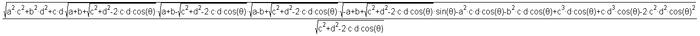SEARCH HOMEMath Central Quandaries & QueriesName: Samuel Status: Student I have a quadrilateral with 4 known sides and 1 known angle, and I'm trying to evaluate the other angles of my quadrilateral. By the law of cosines, I can easily find my opposite angle (using the diagonal as a basis for the equation). However, to find the two remaining angles, I have found no other way so far than to use the other diagonal, which can be found with the equation attached (from geometry atlas). Is there any simpler way?Samuel,

Here is the equation that you sentYou used the Law of Cosines to find the length of a diagonal and then the Law of Cosines again to find the measure of the angle opposite the angle whose measure you were given. Now you have two triangles and for each you know the lengths of the three sides and the measure of one angle. At this point I would use the Law of Sines.

Write back if you still need assistance,
Harley

Say you know AB,BC,CD,DA and the angle a.

The cosine law applied to DAB gives you the diagonal BD.
Either law gives you the other two angles, but the sine law is faster.

The cosine law applied to BCD gives you one of the angles; either law will give you the other two.

You're now pretty close to finished...

Good Hunting!
RDMath Central is supported by the University of Regina and The Pacific Institute for the Mathematical Sciences.